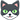# Collection of Science Jokes P2

#### fresh_42

Mentor
2018 Award#### Ibix

They turn out to be an orchestra. If you hadn't guessed.

•davenn

#### DennisN

If you consider buying a decent telescope, the only black hole you will find is the new one in your wallet.

Edit: (I found a picture on the topic)Last edited:
•••Klystron, davenn, BillTre and 1 other person

#### jack action

Gold Member
I love it when human behavior can be expressed mathematically:••Demystifier, Klystron, Wrichik Basu and 1 other person

#### nuuskur

..so that he will be increasing the dosage? (if we forget about the intended derivative joke)

•Wrichik Basu

#### strangerep

I love it when human behavior can be expressed mathematically [...]
Shouldn't there be an integral sign? I.e. $\int\!e^x$

•Klystron

#### davenn

Gold Member••phinds, DrClaude, DennisN and 5 others

#### davenn

Gold Member
I have many chemistry puns,
but I’m afraid they won’t get a good reaction

•DennisN

#### fresh_42

Mentor
2018 Award
I have many chemistry puns,
but I’m afraid they won’t get a good reaction.
On the internet you need a-a-lyst.

•DennisN and davenn

#### Greg Bernhardt••Demystifier, strangerep, davenn and 1 other person

#### jack action

Gold Member••DennisN, Demystifier, davenn and 1 other person

#### davenn

Gold Member••DennisN, BillTre, fresh_42 and 4 others

#### davenn

Gold Member••collinsmark, BillTre, fresh_42 and 3 others

#### Ibix

Dewey decimal? Do we ever!

••davenn and DrClaude

#### jack action

Gold Member•••davenn, mfb, collinsmark and 3 others

#### jack action

Gold Member••DennisN, Keith_McClary, Demystifier and 3 others

#### Demystifier

2018 Award
The Great Unification Theory that unifies physics with electric engineering:
$$i=j$$

••DennisN, strangerep, DrClaude and 2 others

#### Demystifier

2018 Award
Application of the Great Unification Theory above to quantum mechanics. The probability current in quantum mechanics for a particle moving in one dimension is
$$j=\frac{\hbar}{2mi} \left( \psi^*\frac{\partial\psi}{\partial x}-\psi\frac{\partial\psi^*}{\partial x} \right)$$
Using the unification formula above, the current can be written in the electric engineering form
$$i=\frac{\hbar}{2mj} \left( \psi^*\frac{\partial\psi}{\partial x}-\psi\frac{\partial\psi^*}{\partial x} \right)$$

#### mfb

Mentor
Write it as $$i=\frac{\hbar}{2mi} \left( \psi^*\frac{\partial\psi}{\partial x}-\psi\frac{\partial\psi^*}{\partial x} \right)$$ and simplify: $$-1=\frac{\hbar}{2m} \left( \psi^*\frac{\partial\psi}{\partial x}-\psi\frac{\partial\psi^*}{\partial x} \right)$$

#### Demystifier

2018 Award
$$-1=\frac{\hbar}{2m} \left( \psi^*\frac{\partial\psi}{\partial x}-\psi\frac{\partial\psi^*}{\partial x} \right)$$
The left-hand side is real and the right-hand side is imaginary, which is only possible if both sides are zero. Hence the wave function is real (which is compatible with the PBR theorem) and $-1=0$ (which makes sense because one cannot have less than zero particles).

#### fresh_42

Mentor
2018 Award
The Great Unification Theory that unifies physics with electric engineering:
$$i=j$$
Typical pop science chasing for headlines! I have made the effort and had a look in the original paper which this article relies on. And there it is said $i=\mathbf{\hat{j}}$.

•Demystifier

#### mfb

Mentor
Look at Mr. Fancy Hat here.

#### DrGreg

Gold Member
And there it is said $i=\mathbf{\hat{j}}$.
I think that implies $1 = 3$?

•Demystifier

#### chasrob

Gold Member
How do you tell the difference between a teacher and a chemist?

•strangerep

#### davenn

Gold Member••DennisN, strangerep, BillTre and 2 others

"Collection of Science Jokes P2"

### Physics Forums Values

We Value Quality
• Topics based on mainstream science
• Proper English grammar and spelling
We Value Civility
• Positive and compassionate attitudes
• Patience while debating
We Value Productivity
• Disciplined to remain on-topic
• Recognition of own weaknesses
• Solo and co-op problem solving